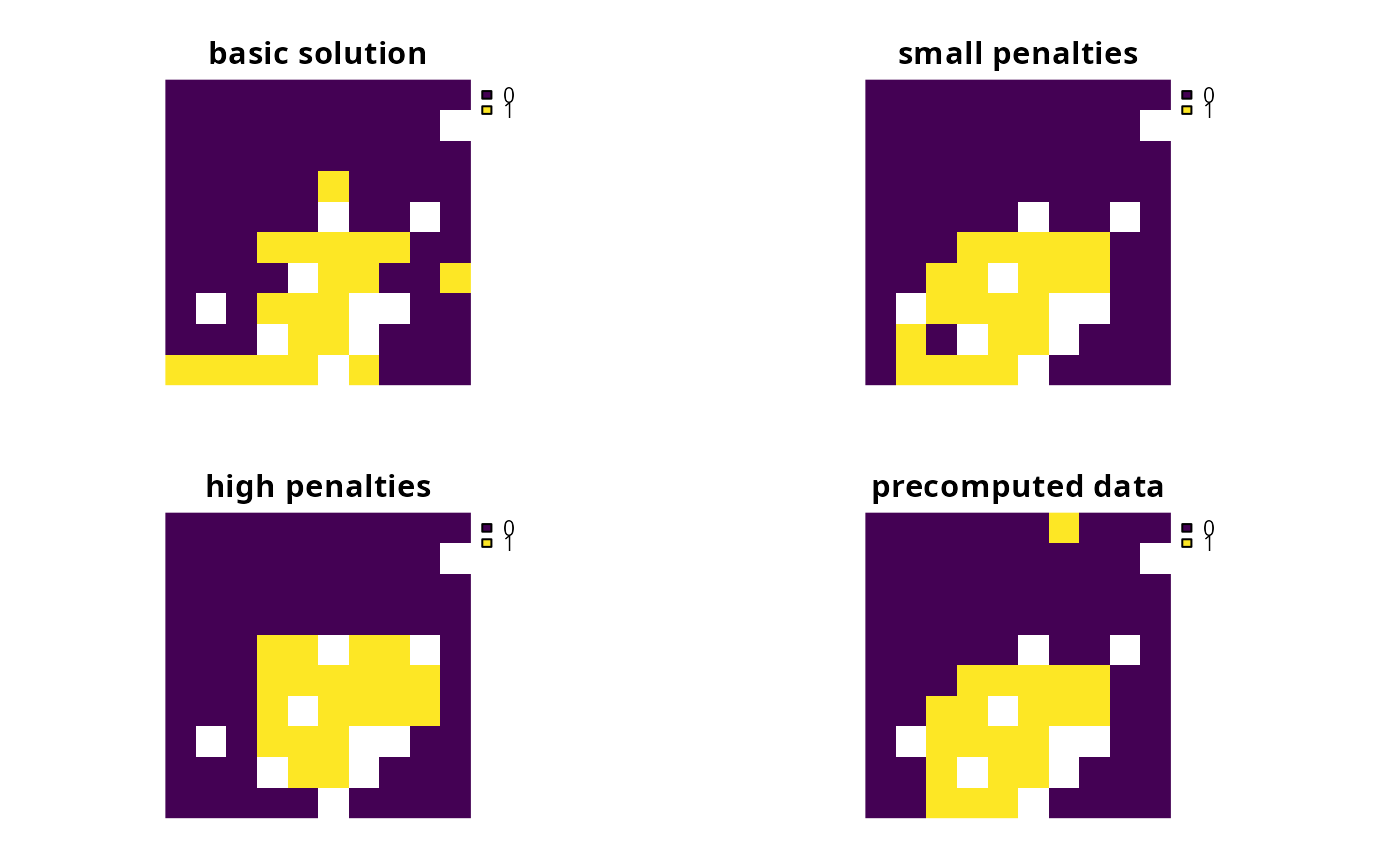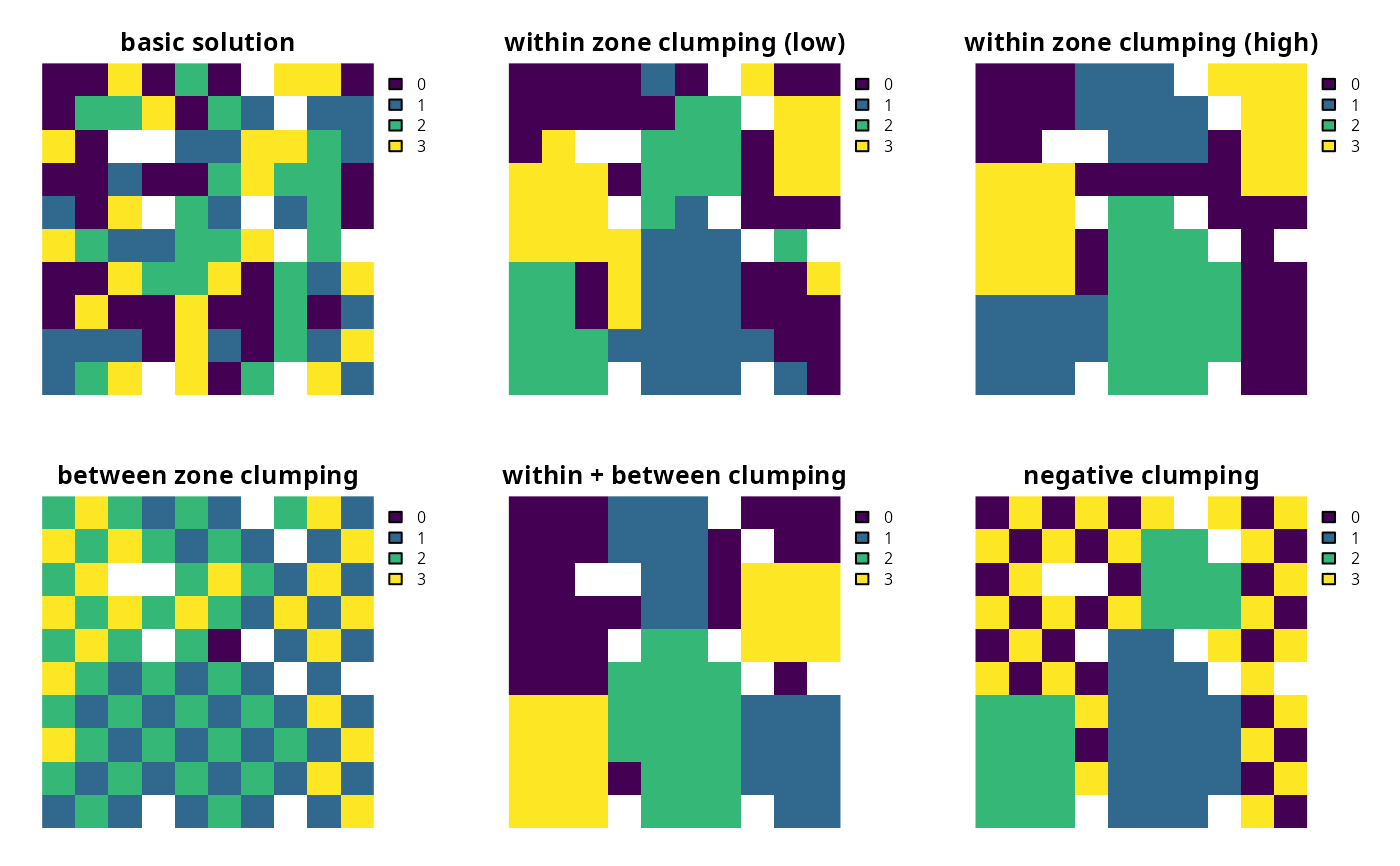Add penalties to a conservation planning problem() to favor solutions that spatially clump planning units together based on the overall boundary length (perimeter).

add_boundary_penalties(
x,
penalty,
edge_factor = rep(0.5, number_of_zones(x)),
zones = diag(number_of_zones(x)),
data = NULL
)

## Arguments

x

problem() (i.e., ConservationProblem) object.

penalty

numeric penalty that is used to scale the importance of selecting planning units that are spatially clumped together compared to the main problem objective (e.g., solution cost when the argument to x has a minimum set objective per add_min_set_objective()). Higher penalty values prefer solutions with a higher degree of spatial clumping, and smaller penalty values prefer solutions with a smaller degree of clumping. Note that negative penalty values prefer solutions that are more spread out. This parameter is equivalent to the boundary length modifier (BLM) parameter in Marxan.

edge_factor

numeric proportion to scale planning unit edges (borders) that do not have any neighboring planning units. For example, an edge factor of 0.5 is commonly used to avoid overly penalizing planning units along a coastline. Note that this argument must have an element for each zone in the argument to x.

zones

matrix or Matrix object describing the clumping scheme for different zones. Each row and column corresponds to a different zone in the argument to x, and cell values indicate the relative importance of clumping planning units that are allocated to a combination of zones. Cell values along the diagonal of the matrix represent the relative importance of clumping planning units that are allocated to the same zone. Cell values must range between 1 and -1, where negative values favor solutions that spread out planning units. The default argument to zones is an identity matrix (i.e., a matrix with ones along the matrix diagonal and zeros elsewhere), so that penalties are incurred when neighboring planning units are not assigned to the same zone. If the cells along the matrix diagonal contain markedly smaller values than those found elsewhere in the matrix, then solutions are preferred that surround planning units with those allocated to different zones (i.e., greater spatial fragmentation).

data

NULL, data.frame, matrix, or Matrix object containing the boundary data. These data describe the total amount of boundary (perimeter) length for each planning unit, and the amount of boundary (perimeter) length shared between different planning units (i.e., planning units that are adjacent to each other). See the Data format section for more information.

## Value

Object (i.e., ConservationProblem) with the penalties added to it.

## Details

This function adds penalties to a conservation planning problem to penalize fragmented solutions. It was is inspired by Ball et al. (2009) and Beyer et al. (2016). The penalty argument is equivalent to the boundary length modifier (BLM) used in Marxan. Note that this function can only be used to represent symmetric relationships between planning units. If asymmetric relationships are required, use the add_connectivity_penalties() function.

## Data format

The argument to data can be specified using the following formats. Note that boundary data must always describe symmetric relationships between planning units.

data as a NULL value

indicating that the data should be automatically calculated using the boundary_matrix() function. This argument is the default. Note that the boundary data must be supplied using one of the other formats below if the planning unit data in the argument to x do not explicitly contain spatial information (e.g., planning unit data are a data.frame or numeric class).

data as a matrix/Matrix object

where rows and columns represent different planning units and the value of each cell represents the amount of shared boundary length between two different planning units. Cells that occur along the matrix diagonal represent the amount of exposed boundary associated with each planning unit that has no neighbor (e.g., these value might pertain to boundaries along a coastline).

data as a data.frame object

with the columns "id1", "id2", and "boundary". The "id1" and "id2" columns contain identifiers (indices) for a pair of planning units, and the "boundary" column contains the amount of shared boundary length between these two planning units. This format follows the the standard Marxan format for boundary data (i.e., per the "bound.dat" file).

## Mathematical formulation

The boundary penalties are implemented using the following equations. Let $$I$$ represent the set of planning units (indexed by $$i$$ or $$j$$), $$Z$$ represent the set of management zones (indexed by $$z$$ or $$y$$), and $$X_{iz}$$ represent the decision variable for planning unit $$i$$ for in zone $$z$$ (e.g., with binary values one indicating if planning unit is allocated or not). Also, let $$p$$ represent the argument to penalty, $$E_z$$ represent the argument to edge_factor, $$B_{ij}$$ represent the matrix argument to data (e.g., generated using boundary_matrix()), and $$W_{zz}$$ represent the matrix argument to zones.

$$\sum_{i}^{I} \sum_{j}^{I} \sum_{z}^{Z} (\mathit{ifelse}(i == j, E_z, 1) \times p \times W_{zz} B_{ij}) + \sum_{i}^{I} \sum_{j}^{I} \sum_{z}^{Z} \sum_{y}^{Z} (-2 \times p \times X_{iz} \times X_{jy} \times W_{zy} \times B_{ij})$$

Note that when the problem objective is to maximize some measure of benefit and not minimize some measure of cost, the term $$p$$ is replaced with $$-p$$.

See penalties for an overview of all functions for adding penalties.

Other penalties: add_asym_connectivity_penalties(), add_connectivity_penalties(), add_feature_weights(), add_linear_penalties()

## Examples

# \dontrun{
# set seed for reproducibility
set.seed(500)

data(sim_pu_raster, sim_features, sim_pu_zones_stack, sim_features_zones)

# create minimal problem
p1 <- problem(sim_pu_raster, sim_features) %>%

# create problem with low boundary penalties
p2 <- p1 %>% add_boundary_penalties(50, 1)

# create problem with high boundary penalties but outer edges receive
# half the penalty as inner edges
p3 <- p1 %>% add_boundary_penalties(500, 0.5)

# create a problem using precomputed boundary data
bmat <- boundary_matrix(sim_pu_raster)
p4 <- p1 %>% add_boundary_penalties(50, 1, data = bmat)

# solve problems
s <- stack(solve(p1), solve(p2), solve(p3), solve(p4))

# plot solutions
plot(s, main = c("basic solution", "small penalties", "high penalties",
"precomputed data"), axes = FALSE, box = FALSE)# create minimal problem with multiple zones and limit the run-time for
# solver to 10 seconds so this example doesn't take too long
p5 <- problem(sim_pu_zones_stack, sim_features_zones) %>%
add_relative_targets(matrix(0.2, nrow = 5, ncol = 3)) %>%
add_default_solver(time_limit = 10, verbose = FALSE)

# create zone matrix which favors clumping planning units that are
# allocated to the same zone together - note that this is the default
zm6 <- diag(3)
print(zm6)
#>      [,1] [,2] [,3]
#> [1,]    1    0    0
#> [2,]    0    1    0
#> [3,]    0    0    1

# create problem with the zone matrix and low penalties
p6 <- p5 %>% add_boundary_penalties(50, zone = zm6)

# create another problem with the same zone matrix and higher penalties
p7 <- p5 %>% add_boundary_penalties(500, zone = zm6)

# create zone matrix which favors clumping units that are allocated to
# different zones together
zm8 <- matrix(1, ncol = 3, nrow = 3)
diag(zm8) <- 0
print(zm8)
#>      [,1] [,2] [,3]
#> [1,]    0    1    1
#> [2,]    1    0    1
#> [3,]    1    1    0

# create problem with the zone matrix
p8 <- p5 %>% add_boundary_penalties(500, zone = zm8)

# create zone matrix which strongly favors clumping units
# that are allocated to the same zone together. It will also prefer
# clumping planning units in zones 1 and 2 together over having
# these planning units with no neighbors in the solution
zm9 <- diag(3)
zm9[upper.tri(zm9)] <- c(0.3, 0, 0)
zm9[lower.tri(zm9)] <- zm9[upper.tri(zm9)]
print(zm9)
#>      [,1] [,2] [,3]
#> [1,]  1.0  0.3    0
#> [2,]  0.3  1.0    0
#> [3,]  0.0  0.0    1

# create problem with the zone matrix
p9 <- p5 %>% add_boundary_penalties(500, zone = zm9)

# create zone matrix which favors clumping planning units in zones 1 and 2
# together, and favors planning units in zone 3 being spread out
# (i.e., negative clumping)
zm10 <- diag(3)
zm10[3, 3] <- -1
print(zm10)
#>      [,1] [,2] [,3]
#> [1,]    1    0    0
#> [2,]    0    1    0
#> [3,]    0    0   -1

# create problem with the zone matrix
p10 <- p5 %>% add_boundary_penalties(500, zone = zm10)

# solve problems
s2 <- stack(category_layer(solve(p5)), category_layer(solve(p6)),
category_layer(solve(p7)), category_layer(solve(p8)),
category_layer(solve(p9)), category_layer(solve(p10)))

# plot solutions
plot(s2, main = c("basic solution", "within zone clumping (low)",
"within zone clumping (high)", "between zone clumping",
"within + between clumping", "negative clumping"),
axes = FALSE, box = FALSE)# }